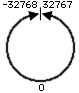KNOWLEDGE BASE
Tech Tip: Integers and number overflow
PRODUCT: 4D | VERSION: | PLATFORM: Mac & Win
Published On: June 2, 2000

The integer type in 4D can hold a number between -32,768 and 32,767. If a number is entered into an integer that is greater than or less than these limits, the number will be modified to fit within the limits.This conversion process is called overflow. For example, if you add 1 to the integer 32,767, it will return -32768. If you subtract 1 from the integer -32,768, you will get 32,767. The picture below illustrates how 4D will store the integer using overflow. Numbers greater than 32,767 are converted directly into negative numbers and vice versa.The other fact to know about integers is that an integer field and an integer array are in fact integers. An integer variable is really a long integer. This has been true since at least version 3.

To explore this for yourself, cut and paste the following code example and run in a debugger:

CREATE RECORD([Table1])
[Table1]Int:=32766
[Table1]Int:=[Table1]Int+1 ` 32,767
[Table1]Int:=[Table1]Int+1 ` -32,768
[Table1]Int:=[Table1]Int-1 ` 32,767

ARRAY INTEGER(aInteger;1)
aInteger{1}:=32766
aInteger{1}:=aInteger{1}+1 ` 32,767
aInteger{1}:=aInteger{1}+1 ` -32,768

C_INTEGER(vInt)
vInt:=32766
vInt:=vInt+1 ` 32,767
vInt:=vInt+1 ` ! 32,768 !

If (Type(vInt)=Is LongInt )